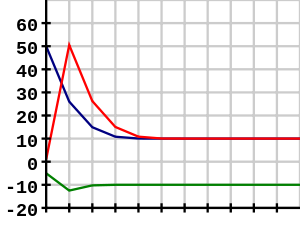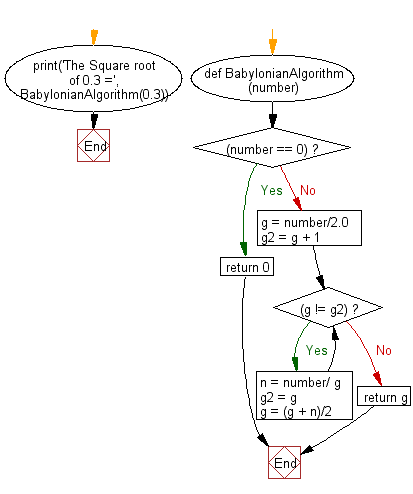﻿ Python Math: Computing square roots using the Babylonian method - w3resource# Python Math: Computing square roots using the Babylonian method

## Python Math: Exercise-18 with Solution

Write a Python program to computing square roots using the Babylonian method.

Perhaps the first algorithm used for approximating √S is known as the Babylonian method, named after the Babylonians, or "Hero's method", named after the first-century Greek mathematician Hero of Alexandria who gave the first explicit description of the method. It can be derived from (but predates by 16 centuries) Newton's method. The basic idea is that if x is an overestimate to the square root of a non-negative real number S then S / x will be an underestimate and so the average of these two numbers may reasonably be expected to provide a better approximation.

Graph PresentationGraph charting the use of the Babylonian method for approximating the square root of 100 (10) using starting values x0 = 50, x0 = 1, and x0 = −5. Note that using a negative starting value yields the negative root.
Graph Credits: Crotalus Horridus

Sample Solution:-

Python Code:

``````def BabylonianAlgorithm(number):
if(number == 0):
return 0;

g = number/2.0;
g2 = g + 1;
while(g != g2):
n = number/ g;
g2 = g;
g = (g + n)/2;

return g;
print('The Square root of 0.3 =', BabylonianAlgorithm(0.3));
```
```

Sample Output:

```The Square root of 0.3 = 0.5477225575051661
```

Flowchart:## Visualize Python code execution:

The following tool visualize what the computer is doing step-by-step as it executes the said program:

Python Code Editor:

Have another way to solve this solution? Contribute your code (and comments) through Disqus.

What is the difficulty level of this exercise?

Test your Programming skills with w3resource's quiz.

﻿

```>>> students = [{'name': 'John', 'score': 98}, {'name': 'Mike', 'score': 94}, {'name': 'Jennifer', 'score': 99}]